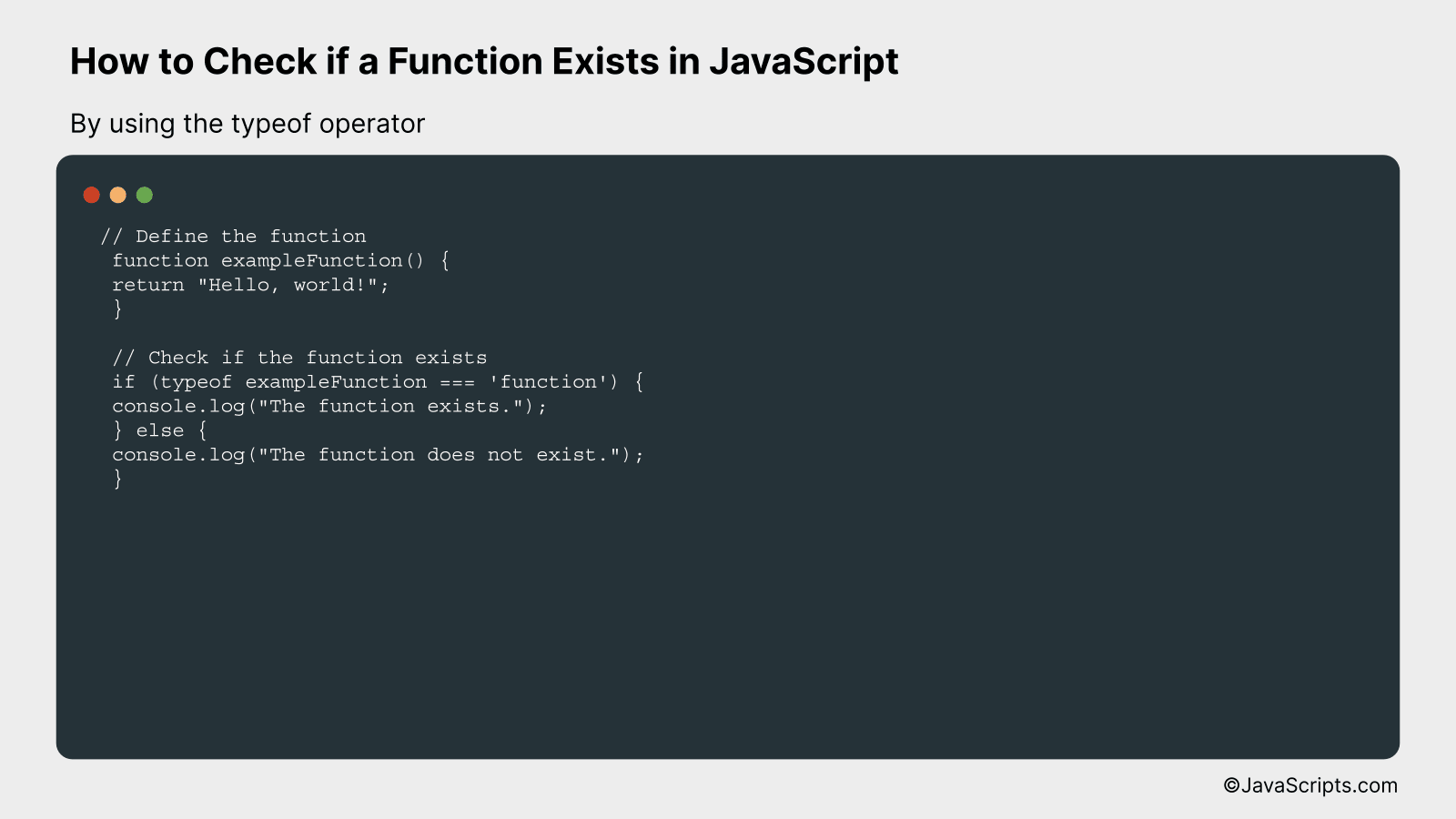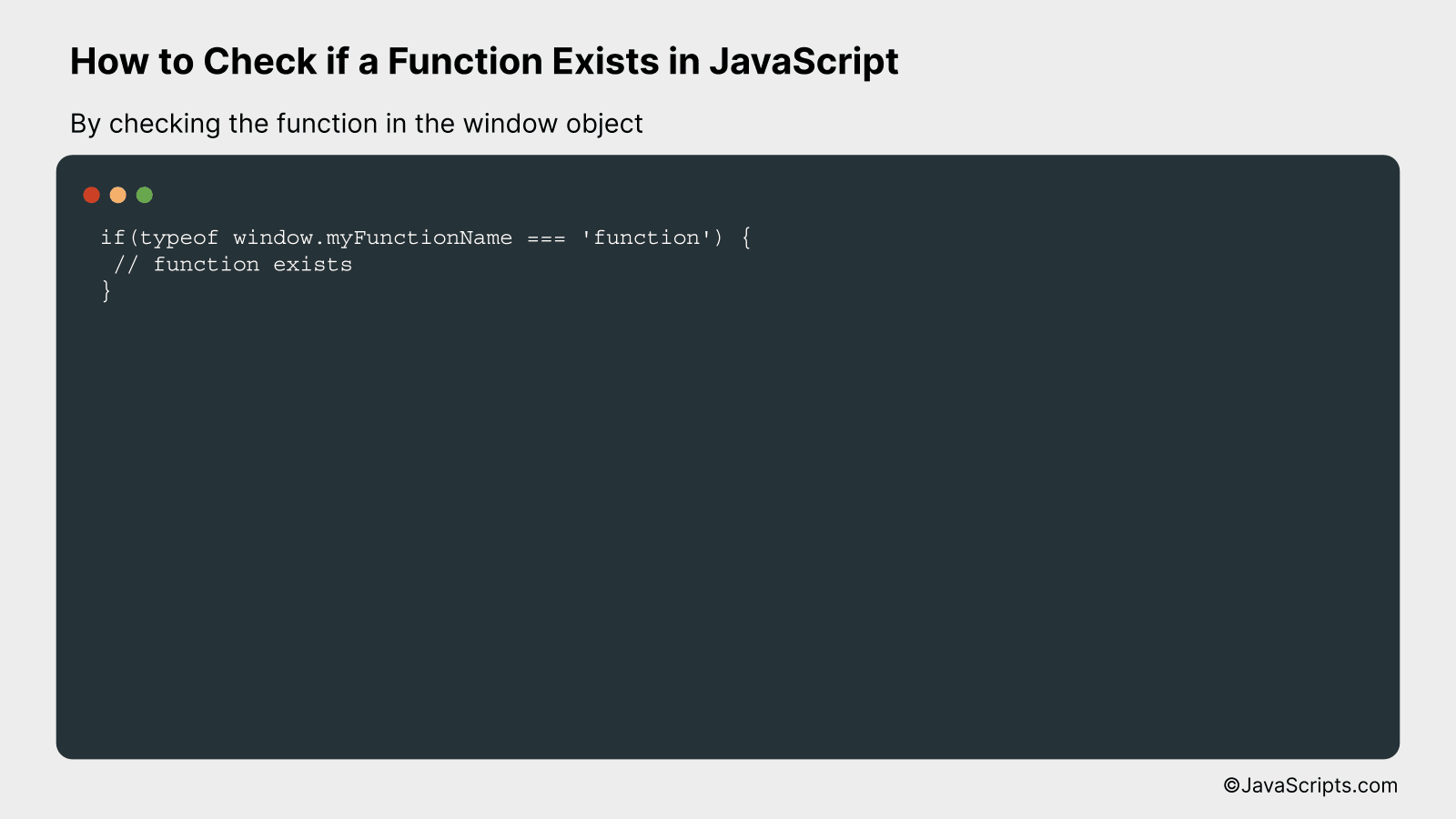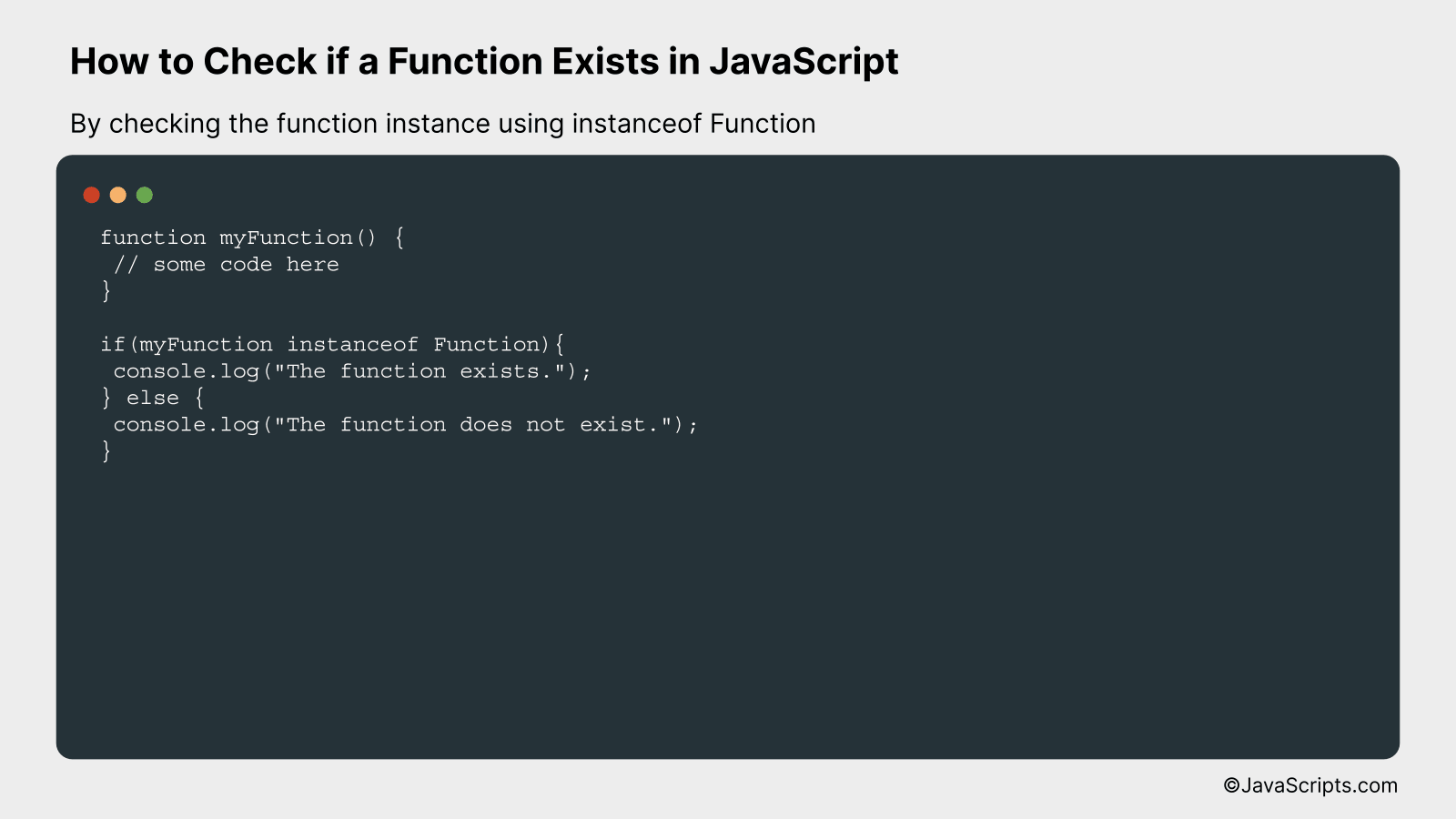# How to Check if a Function Exists in JavaScript

Ever faced a situation where you’re not sure whether a function exists in your JavaScript code? You’re not alone. This is a common scenario many programmers encounter, especially when working with large codes or when using scripts from other developers.

So, how can we tackle this issue? It’s simple. There are several effective ways to check if a function exists in JavaScript. Let’s dive deeper into this together and shed light on these methods to make your coding process a breeze.

In this post, we will be looking at the following 3 ways to check if a function exists in JavaScript:

• By using the typeof operator
• By checking the function in the window object
• By checking the function instance using instanceof Function

Let’s explore each…

## #1 – By using the typeof operatorBy using the ‘typeof’ operator in JavaScript, you can check if a function exists. This operator returns a string indicating the type of the evaluated operand. If the function exists, ‘typeof’ will return ‘function’; otherwise, it will return ‘undefined’. This method of checking function existence can be understood better with an example.

``````
// Define the function
function exampleFunction() {
return "Hello, world!";
}

// Check if the function exists
if (typeof exampleFunction === 'function') {
console.log("The function exists.");
} else {
console.log("The function does not exist.");
}
```
```

### How it works

The ‘typeof’ operator in JavaScript is used to check the data type of its operand. Here we are using it to check if a certain function (in this case, ‘exampleFunction’) exists or not.

This works by the following steps:

• We declare a function ‘exampleFunction’.
• We then use the ‘typeof’ operator to evaluate the type of ‘exampleFunction’.
• If ‘exampleFunction’ exists and is indeed a function, ‘typeof exampleFunction’ will return ‘function’.
• We then compare this return value with the string ‘function’ using the strict equality operator ‘===’.
• If the comparison is true, which means ‘exampleFunction’ exists and is a function, we print “The function exists.” to the console.
• If the comparison is false, which means ‘exampleFunction’ does not exist or is not a function, we print “The function does not exist.” to the console.

## #2 – By checking the function in the window objectWe will be checking if a function exists in JavaScript by looking into the window object. This can be understood better with an example. The window object in JavaScript is a global object that holds variables, functions, history, location, and many more global information. We can use this object to check if a function is declared globally in the window.

``````
if(typeof window.myFunctionName === 'function') {
// function exists
}
``````

### How it works

The above statement is a condition that checks if a function named ‘myFunctionName’ exists in the window object. The ‘typeof’ operator is used to get the type of the object. If ‘myFunctionName’ is a function, ‘typeof’ returns ‘function’. If the function does not exist, ‘typeof’ returns ‘undefined’, and the condition fails.

• First, we access the function by its name from the window object using ‘window.myFunctionName’.
• Then we use the ‘typeof’ operator to get the type of this object. If the function exists, it will return ‘function’. If it doesn’t exist, it will return ‘undefined’.
• Finally, we compare the returned type with the string ‘function’. If they are the same, it means the function exists. If they are different, it means the function doesn’t exist.

## #3 – By checking the function instance using instanceof FunctionThis method allows you to check if a specific function exists in JavaScript by verifying if its instance is a type of Function. You can understand it better by looking at an example where we define a function and then use “instanceof Function” to check if it exists.

``````
function myFunction() {
// some code here
}

if(myFunction instanceof Function){
console.log("The function exists.");
} else {
console.log("The function does not exist.");
}
``````

### How it works

The keyword ‘instanceof’ in JavaScript is used to check the type of an object. When used with ‘Function’, it checks if the specified object is a function.

• The ‘myFunction’ is first defined as a function in the JavaScript code.
• We then use an ‘if’ statement combined with ‘instanceof Function’ to check if ‘myFunction’ is a function.
• If ‘myFunction’ is a function, it returns true, and “The function exists.” is logged to the console.
• If ‘myFunction’ is not a function, it returns false, and “The function does not exist.” is logged to the console.

Related:

In conclusion, it’s essential to understand how to verify the existence of a function in JavaScript before invoking it. This helps in avoiding errors that could disrupt the user’s experience or cause your program to crash.

The ‘typeof’ operator is a simple and effective tool for this task. By checking if the type of a function is ‘function’, you can ascertain whether it exists or not. This practice aids in writing cleaner, more error-resistant code.